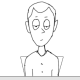# Game Development in Android using Libgdx Part VII

In this tutorial we will start building the class for the protagonist. The protagonist went through many name changes in our game but for the  purpose of this tutorial it would be called Hero. Its shape would be circular so from the physics perspective we will make a class to handle circular objects collisions with others.

BaseObject class is defined in Last Tutorial (Game Development in Android using Libgdx Part VI).

```public class CircleObject extends BaseObject{
public Boolean IsSensor;
public Vector2 Velocity;
public float Mass;   //For static bodies this is set to 0
public CircleObject(float r){
IsSensor=false;
Velocity=new Vector2();
}
public void Update(float dt){
if(IsActive){
Position.x=Position.x+Velocity.x*dt;
Position.y=Position.y+Velocity.y*dt;
}
}
}```

So CircleObject is a physics object which will hold the position,velocity and radius of the circle. IsSensor is used to check if response of a collision with other objects has to be handled. This property is useful while colliding with something like health, a star or anything which should not change/affect the motion of the object. So if IsSensor is true then no response is initiated we just handle the event of collision.

CollisionHandler.java

```public class CollisionHandler{
public static Vector2 Direction=new Vector2();
public static Vector2 MoveDX=new Vector2();
public static Vector2 RelativeVelocity=new Vector2();
public static float e=1f;
public boolean CheckCollision(CircleObject obj1,CircleObject obj2){
float distanceSquare=obj1.Position.dst2(obj2.Position); //dst2 Gives square of distance
if(!obj1.IsSensor && !obj2.IsSensor){
//HANDLE COLLISION RESPONSE
CollisionResponse(obj1,obj2);
}
}
}
static void CollisionResponse(CircleObject obj1,CircleObject obj2){
Direction.set(obj1.Position.x-obj2.Position.x,obj1.Position.y-obj2.Position.y);
Direction.nor();  //Normalizes the tangent so its magnitude=1
float obj1MassRatio=obj1.Mass/(obj1.Mass+obj2.Mass);
float obj2MassRatio=obj2.Mass/(obj1.Mass+obj2.Mass);
MoveDX.set(-overlapDistance*obj1MassRatio*Direction.x,-overlapDistance*obj1MassRatio*Direction.y);
MoveDX.set(overlapDistance*obj2MassRatio*Direction.x,overlapDistance*obj2MassRatio*Direction.y);
RelativeVelocity.set(obj1.Velocity.x-obj2.Velocity.x,obj1.Velocity.y-obj2.Velocity.y);
float dotVelocity=RelativeVelocity.dot(Direction)*2*e;
obj1.Velocity.sub(Direction.mul(dot*obj1MassRatio));
}

}```

CollisionHandler is going to handle collisions between different objects of the game. Currently we just handled the collision of a circleobject with another circleobject. For response we check if both are non sensor objects and the ratio of the movement of the bodies depends on the mass. For static bodies we put mass as “0”.  On collision e is the coefficient of how elastic the collision is. Setting e as 1 makes collision completely elastic. Though for our game polar there was never a case when on collision both bodies would be dynamic and on response both will remain alive (in the later levels we have projectiles which are shot in specific directions and collision check is done for them with the player but these projectiles are deleted before the next iteration). For the direction we take the vector2 pointing from one object’s to center to other. So for one body the distance is added and other it is subtracted.

Hero.java

```public class Hero extends CircleObject{
public TextureWrapper HeroTexture;
Position=pos;
HeroTexture=new TextureWrapper(texRegion,pos);
}
public void Draw(SpriteBatch sp){
if(IsActive)
HeroTexture.Draw(sp);
}
public void Update(float dt){
super.Update(dt);
}

public void ApplyImpulse(Vector2 impulse){
}

}```

More features would be added to this class later on but for now we are making our hero a circular object. We give a texture to be drawn to show it on the screen. CircleObject on its own does not have any texture to show on the screen so we attach a texture here. Update just updates its position for now. ApplyImpulse is to handle the gravity which is applied by the tapping of the finger near the object. And as such, there is no friction or constant gravity in our environment so the velocity remains constant unless collisions/external force is applied.

In the initialize part of the gameplay screen we will just initialize our hero. We have to pass the radius, texture Region and position to it. We kept Radius and texture as static in a single class which we used to tinker with from time to time so see what fits best. Keeping them in one place helps to manage changes easily. For the level mode, we loaded the position from the text file that we stored for each level. For infinite time mode we just randomize the position.

We made physics objects and added collision checking for them but to handle all of these objects updates and collisions we would have to make a class which keeps all of them in one place (mostly in an array).

ObjectManager.java

```public class ObjectManager{
public int freeIndex;
public BaseObject[] objects;
public static final int INIT_ARRAY_SIZE=20;
public static final int ARRAY_SIZE_INC=20;
static ObjectManager instance;
ObjectManager(){
objects=new BaseObject[INIT_ARRAY_SIZE];
}
public static ObjectManager GetInstance(){
if(instance==null){
instance=new ObjectManager();
}
return instance;
}
objects[freeIndex]=obj;
objects[freeIndex].UniqueId=freeIndex;
freeIndex++;
//CHECK if array size increases the limit then extend the array
if(freeIndex==objects.length){
BaseObject[] temp=new BaseObject[objects.length+ARRAY_SIZE_INC];
for(int i=0;i<freeIndex;i++){
temp[i]=objects[i];
objects[i]=null;
}
objects=temp;
}
}
public void RemoveObject(int index){
objects[index].Destroy();
for(int i=index;i<freeIndex-1;i++){
objects[i+1].UniqueID=objects[i].UniqueID;
objects[i]=objects[i+1];
}
objects[freeIndex]=null;
freeIndex--;
}
public void Update(float dt){
for(int i=0;i<freeIndex;i++){
objects[i].Update(dt);
}
//Check for collisions between bodies and handle respective response
}
}```

This class is used to store all the objects created in the level and handle their updates and collisions. Whenever we create an object we just add it to the ObjectManager. On collisions if there is need to remove an object we just call RemoveObject function with its id.

```//Adding hero to the object manager
ObjectManager objManager=ObjectManager.GetInstance();

So in this tutorial we created our hero, added a shape to it, made a function to check for collisions and made a class to handle all the objects in the game.

Next Tutorial:

In next tutorial we will add the feature to move the Hero on tapping near it.

Thank you for the patience.

Tagged with: , , , ,
###### 2 comments on “Game Development in Android using Libgdx Part VII”
1.Paulo says:

class CircleObject extends BaseGameObject ????

Where’s define BaseGameObject ?

•Rahul Srivastava says:

Hi,

Thanks for pointing out the Typo. It would be BaseObject instead of BaseGameObject which is defined in the last tutorial.

Thanks